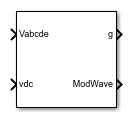# PWM Generator (Five-phase, Two-level)

Generate five-phase, two-level pulse width modulated waveform

• Library:
• Simscape / Electrical / Control / Pulse Width Modulation

•## Description

The PWM Generator (Five-phase, Two-level) block controls switching behavior for a five-phase, two-level power converter. The block:

1. Calculates on-gating and off-gating times based on the block inputs:

• Five sinusoidal reference voltages, one per phase

2. Uses the gating times to generate ten switch-controlling pulses.

3. Uses the gating times to generate modulation waveforms.

### Continuous PWM

The block only provides continuous pulse width modulation (PWM). The figure shows the general difference between continuous sinusoidal pulse width modulation (SPWM) and continuous space vector modulation (SVM) waveforms.### Sampling Mode

This block allows you to choose natural, symmetric, or asymmetric sampling of the modulation wave.

The PWM Generator (Five-phase, Two-level) block does not perform carrier-based PWM. Instead, the block uses input signals to calculate gating times and then uses the gating times to generate both the switch-controlling pulses and the modulation waveforms that it outputs.

Carrier-based PWM is, however, useful for showing how the sampling mode that you select relates to the switch-on and switch-off behavior of the pulses that the block generates. A generator that uses a two-level, carrier-based PWM method:

1. Samples a reference wave.

2. Compares the sample to a triangle carrier wave.

3. Generates a switch-on pulse if a sample is higher than the carrier signal or a switch-off pulse if a sample is lower than the carrier wave.

To determine switch-on and switch-off pulse behavior, a two-level carrier-based PWM generator uses these methods to sample the triangle wave:

• Natural — The sampling and comparison occur at the intersection points of the modulation wave and the carrier wave.• Asymmetric — Sampling occurs at the upper and lower boundaries of the carrier wave. The comparison occurs at the intersection that follows the sampling.• Symmetric — Sampling occurs at only the upper boundary of the carrier wave. The comparison occurs at the intersection that follows the sampling.## Ports

### Input

expand all

Sinusoidal voltages that you want the attached converter to output, specified as a vector of five elements with one element for each phase.

Positive real number for the DC-link voltage of the converter, specified as a scalar.

### Output

expand all

Ten pulse waveforms that determine the switching behavior in the attached power converter, returned as a vector.

Modulation waves, returned as a vector of five elements, with one element for each phase.

## Parameters

expand all

Continuous pulse width modulation method.

Wave-sampling method. The sampling mode determines whether the block samples the modulation waveform when the waves intersect or when the carrier wave is at one or both of its boundary conditions.

Rate at which the switches in the power converter switch, in Hz.

Time interval between successive block executions, in seconds. To ensure adequate resolution in the generated signal, set this value to be less than or equal to `1/(50*Fsw)`, where Fsw is the value of the Switching frequency (Hz) parameter.

## Version History

Introduced in R2021a